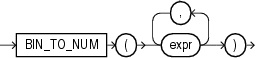# BIN_TO_NUM

SyntaxDescription of the illustration bin_to_num.gif

Purpose

`BIN_TO_NUM` converts a bit vector to its equivalent number. Each argument to this function represents a bit in the bit vector. This function takes as arguments any numeric datatype, or any nonnumeric datatype that can be implicitly converted to `NUMBER`. Each `expr` must evaluate to 0 or 1. This function returns Oracle `NUMBER`.

`BIN_TO_NUM` is useful in data warehousing applications for selecting groups of interest from a materialized view using grouping sets.

Examples

The following example converts a binary value to a number:

```SELECT BIN_TO_NUM(1,0,1,0) FROM DUAL;

BIN_TO_NUM(1,0,1,0)
-------------------
10
```

The next example converts three values into a single binary value and uses `BIN_TO_NUM` to convert that binary into a number. The example uses a PL/SQL declaration to specify the original values. These would normally be derived from actual data sources.

```SELECT order_status FROM orders WHERE order_id = 2441;

ORDER_STATUS
------------
5
DECLARE
warehouse NUMBER := 1;
ground    NUMBER := 1;
insured   NUMBER := 1;
result    NUMBER;
BEGIN
SELECT BIN_TO_NUM(warehouse, ground, insured) INTO result FROM DUAL;
UPDATE orders SET order_status = result WHERE order_id = 2441;
end;
/
PL/SQL procedure successfully completed.

SELECT order_status FROM orders WHERE order_id = 2441;

ORDER_STATUS
------------
7
```

Refer to the examples for BITAND for information on reversing this process, extracting multiple values from a single column value.